# How to do Fuzzy Matching on Pandas Dataframe Column Using Python?

• Difficulty Level : Medium
• Last Updated : 30 May, 2021

Prerequisite: FuzzyWuzzy

In this tutorial, we will learn how to do fuzzy matching on the pandas DataFrame column using Python. Fuzzy matching is a process that lets us identify the matches which are not exact but find a given pattern in our target item. Fuzzy matching is the basis of search engines. That is why we get many recommendations or suggestions as we type our search query in any browser.

### Functions Used

• pd.DataFrame(dict): To convert a python dictionary to pandas dataframe
• dataframe[‘column_name’].tolist(): To convert a particular column of pandas data-frame into a list of items in python
• append(): To append items to a list
• process.extract(query, choice, limit): A function that comes with the processing module of fuzzywuzzy library to extract those items from the choice list which match the given query. The number of the closest choices that are extracted is determined by the limit set by us.
• process.extractOne(query, choice, scorer): Extracts the only closest match from the choice list which matches the given query and scorer is the optional parameter to make it use a particular scorer like fuzz.token_sort_ratio, fuzz.token_set_ratio
• fuzz.ratio: To calculate the similarity ratio between two strings based on Levenshtein distance
• fuzz.partial_ratio: To calculate the partial string ratio between the smallest string against all the n length sub-string of the long string
• fuzz.token_sort_ratio: Calculates the similarity ratio after sorting the tokens in each string
• fuzz.token_set_ratio: It tries to rule out differences in the strings, it returns the maximum ratio after calculating the ratio on three particular sub-string sets in python

### Examples

Example 1: (Basic Approach)

• At first, we will create two dictionaries. Then we will convert it into pandas data frames and create two empty lists for storing the matches later than as shown below:

## Python3

 `from` `fuzzywuzzy ``import` `fuzz``from` `fuzzywuzzy ``import` `process``import` `pandas`` ` `dict1 ``=` `{``'name'``: [``"aparna"``, ``"pankaj"``, ``                  ``"sudhir"``, ``"Geeku"``]}`` ` `dict2 ``=` `{``'name'``: [``"aparn"``, ``"arup"``, ``"Pankaj"``,``                  ``"sudhir c"``, ``"Geek"``, ``"abc"``]}`` ` `# converting to pandas dataframes``dframe1 ``=` `pd.DataFrame(dict1)``dframe2 ``=` `pd.DataFrame(dict2)`` ` `# empty lists for storing the``# matches later``mat1 ``=` `[]``mat2 ``=` `[]`` ` `# printing the pandas dataframes``dframe1.show()``dframe2.show()`

dframe1: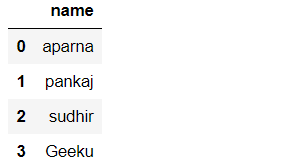dframe2: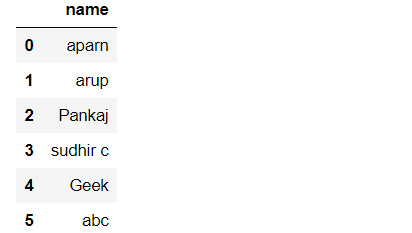• Then we will convert the dataframes into lists using tolist() function.
• We took threshold=80 so that the fuzzy matching occurs only when the strings are at least more than 80% close to each other.

## Python3

 `list1 ``=` `dframe1[``'name'``].tolist()``list2 ``=` `dframe2[``'name'``].tolist()`` ` `# taking the threshold as 80``threshold ``=` `80`

Output: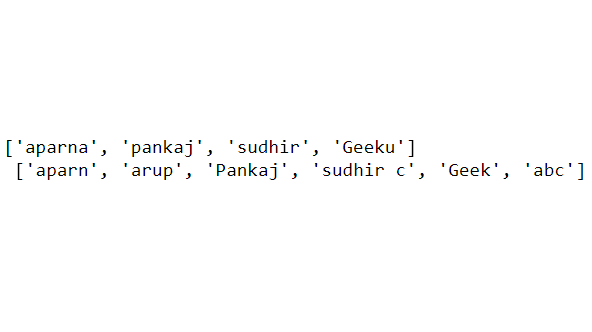• Then we will iterate through the list1 items to extract their closest match from list2.
• Here we use the process.extract() function from the processing module to extract the elements.
• Limit=2 means it will extract the two closest elements with their accuracy ratio, if we print it now then we can see the ratio values.
• Then we append each closest match to the list mat1
• And store the list of matches under column ‘matches’ in the first dataframe i.e dframe1

## Python3

 `# iterating through list1 to extract ``# it's closest match from list2``for` `i ``in` `list1:``    ``mat1.append(process.extract(i, list2, limit``=``2``))``dframe1[``'matches'``] ``=` `mat1`` ` `dframe1.show()`

Output: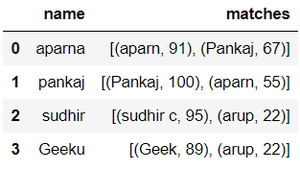• Then we will again iterate through the matches column in the outer loop and in the inner loop we iterate through each set of matches
• k >= threshold means it will select only those items whose threshold value is greater than or equal to 80 and append those to list p.
• Using the “,”.join() function join the item matches are separated by a comma if there are more than one matches for a particular column item and append it to list mat2. We set list p empty again for storing the matches of the next row item in the first dataframe column.
• Then we store the resultant closest matches back to dframe1 to get our final output.

## Python3

 `# iterating through the closest``# matches to filter out the``# maximum closest match``for` `j ``in` `dframe1[``'matches'``]:``    ``for` `k ``in` `j:``       ` `        ``if` `k[``1``] >``=` `threshold:``            ``p.append(k[``0``])``             ` `    ``mat2.append(``","``.join(p))``    ``p ``=` `[]``     ` `# storing the resultant matches ``# back to dframe1``dframe1[``'matches'``] ``=` `mat2`` ` `dframe1.show()`

Output: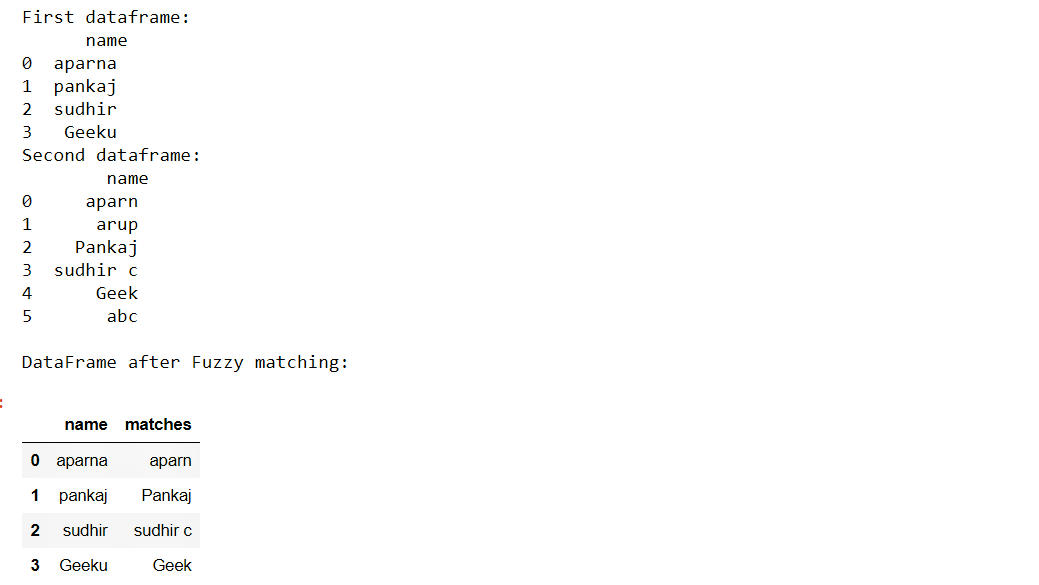Example 2:

In this example, the steps are the same as in example one. Only the difference is that there are multiple matches for a particular row item like for ‘mango’ and ‘choco’. We set the threshold=82 to increase fuzzy match accuracy.

## Python3

 `import` `pandas as pd``from` `fuzzywuzzy ``import` `fuzz``from` `fuzzywuzzy ``import` `process`` ` `# creating the dictionaries``dict1 ``=` `{``'name'``: [``"mango"``, ``"coco"``, ``"choco"``, ``"peanut"``, ``"apple"``]}``dict2 ``=` `{``'name'``: [``"mango fruit"``, ``"coconut"``, ``"chocolate"``,``                  ``"mangoes"``, ``"chocos"``, ``"peanuts"``, ``"appl"``]}`` ` `# converting to pandas dataframes``dframe1 ``=` `pd.DataFrame(dict1)``dframe2 ``=` `pd.DataFrame(dict2)`` ` `# empty lists for storing the matches later``mat1 ``=` `[]``mat2 ``=` `[]``p ``=` `[]`` ` `# printing the pandas dataframes``print``(``"First dataframe:\n"``, dframe1, ``      ``"\nSecond dataframe:\n"``, dframe2)`` ` `# converting dataframe column to list``# of elements``# to do fuzzy matching``list1 ``=` `dframe1[``'name'``].tolist()``list2 ``=` `dframe2[``'name'``].tolist()`` ` `# taking the threshold as 82``threshold ``=` `82`` ` `# iterating through list1 to extract ``# it's closest match from list2``for` `i ``in` `list1:``    ``mat1.append(process.extract(i, list2, limit``=``2``))``dframe1[``'matches'``] ``=` `mat1`` ` `# iterating through the closest matches``# to filter out the maximum closest match``for` `j ``in` `dframe1[``'matches'``]:``    ``for` `k ``in` `j:``        ``if` `k[``1``] >``=` `threshold:``            ``p.append(k[``0``])``    ``mat2.append(``","``.join(p))``    ``p ``=` `[]`` ` ` ` `# storing the resultant matches back to dframe1``dframe1[``'matches'``] ``=` `mat2``print``(``"\nDataFrame after Fuzzy matching:"``)``dframe1`

Output: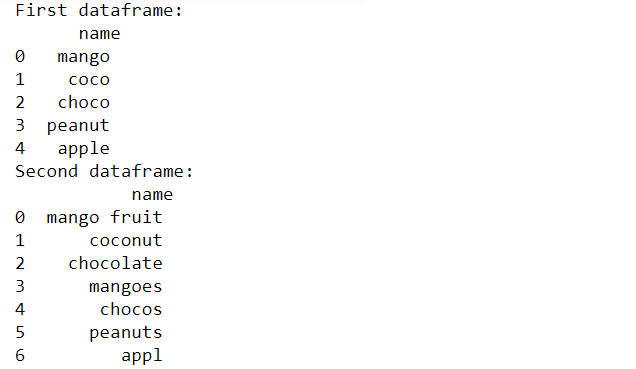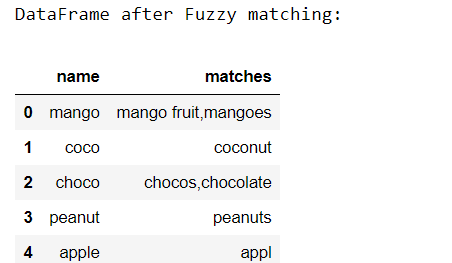Now we will use the process.extractOne() method to match only the closest between the two dataframes. Inside this method we will apply different fuzzy matching functions which are as follows:

Example 3: Using fuzz.ratio()

## Python3

 `import` `pandas as pd``from` `fuzzywuzzy ``import` `fuzz``from` `fuzzywuzzy ``import` `process`` ` `# creating the dictionaries``dict1 ``=` `{``'name'``: [``"aparna"``, ``"pankaj"``, ``"sudhir"``,``                  ``"Geeku"``, ``"geeks for geeks"``]}`` ` `dict2 ``=` `{``'name'``: [``"aparn"``, ``"arup"``, ``"Pankaj"``,``                  ``"for geeks geeks"``, ``"sudhir c"``,``                  ``"geeks geeks"``]}`` ` `# converting to pandas dataframes``dframe1 ``=` `pd.DataFrame(dict1)``dframe2 ``=` `pd.DataFrame(dict2)`` ` `# empty lists for storing the matches ``# later``mat1 ``=` `[]``mat2 ``=` `[]``p ``=` `[]`` ` `# printing the pandas dataframes``print``(``"First dataframe:\n"``, dframe1,``      ``"\nSecond dataframe:\n"``, dframe2)`` ` `# converting dataframe column to ``# list of elements``# to do fuzzy matching``list1 ``=` `dframe1[``'name'``].tolist()``list2 ``=` `dframe2[``'name'``].tolist()`` ` `# taking the threshold as 80``threshold ``=` `80`` ` `# iterating through list1 to extract ``# it's closest match from list2``for` `i ``in` `list1:``    ``mat1.append(process.extractOne(i, list2, scorer``=``fuzz.ratio))``dframe1[``'matches'``] ``=` `mat1`` ` `# iterating through the closest matches``# to filter out the maximum closest match``for` `j ``in` `dframe1[``'matches'``]:``    ``if` `j[``1``] >``=` `threshold:``        ``p.append(j[``0``])``    ``mat2.append(``","``.join(p))``    ``p ``=` `[]`` ` ` ` `# storing the resultant matches back to dframe1``dframe1[``'matches'``] ``=` `mat2``print``(``"\nDataFrame after Fuzzy matching using fuzz.ratio():"``)``dframe1`

Output: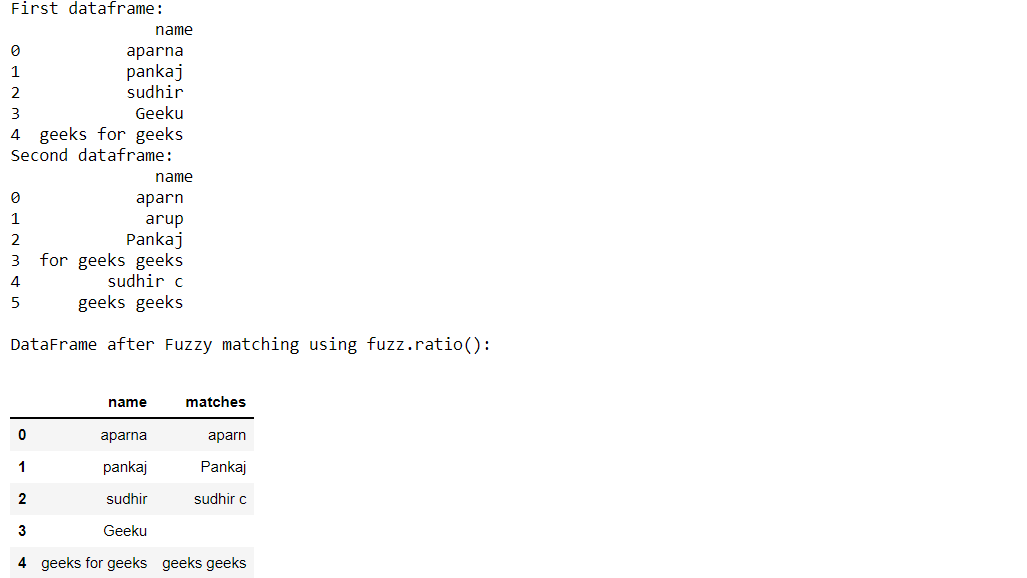Example 4: Using fuzz.partial_ratio()

## Python3

 `import` `pandas as pd``from` `fuzzywuzzy ``import` `fuzz``from` `fuzzywuzzy ``import` `process`` ` `# creating the dictionaries``dict1 ``=` `{``'name'``: [``"aparna"``, ``"pankaj"``, ``"sudhir"``, ``                  ``"Geeku"``, ``"geeks for geeks"``]}`` ` `dict2 ``=` `{``'name'``: [``"aparn"``, ``"arup"``, ``"Pankaj"``,``                  ``"for geeks geeks"``, ``"sudhir c"``, ``                  ``"geeks geeks"``]}`` ` `# converting to pandas dataframes``dframe1 ``=` `pd.DataFrame(dict1)``dframe2 ``=` `pd.DataFrame(dict2)`` ` `# empty lists for storing the matches ``# later``mat1 ``=` `[]``mat2 ``=` `[]``p ``=` `[]`` ` `# printing the pandas dataframes``print``(``"First dataframe:\n"``, dframe1,``      ``"\nSecond dataframe:\n"``, dframe2)`` ` `# converting dataframe column to ``# list of elements``# to do fuzzy matching``list1 ``=` `dframe1[``'name'``].tolist()``list2 ``=` `dframe2[``'name'``].tolist()`` ` `# taking the threshold as 80``threshold ``=` `80`` ` `# iterating through list1 to extract ``# it's closest match from list2``for` `i ``in` `list1:``    ``mat1.append(process.extractOne(``      ``i, list2, scorer``=``fuzz.partial_ratio))``dframe1[``'matches'``] ``=` `mat1`` ` `# iterating through the closest matches``# to filter out the maximum closest match``for` `j ``in` `dframe1[``'matches'``]:``    ``if` `j[``1``] >``=` `threshold:``        ``p.append(j[``0``])``    ``mat2.append(``","``.join(p))``    ``p ``=` `[]`` ` ` ` `# storing the resultant matches back to dframe1``dframe1[``'matches'``] ``=` `mat2``print``(``"\nDataFrame after Fuzzy matching using fuzz.partial_ratio:"``)``dframe1`

Output: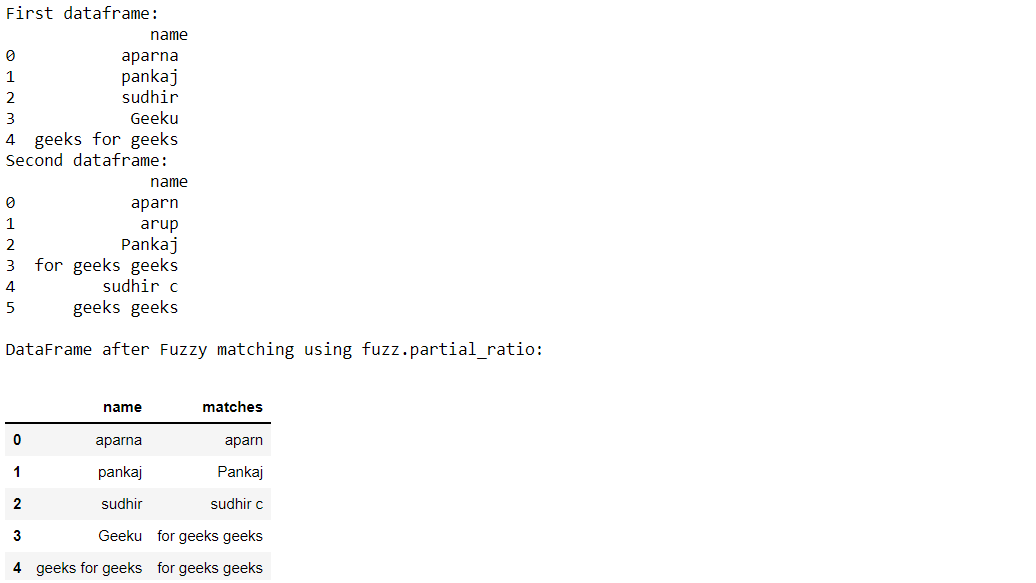Example 5: Using fuzz.token_sort_ratio()

## Python3

 `import` `pandas as pd``from` `fuzzywuzzy ``import` `fuzz``from` `fuzzywuzzy ``import` `process`` ` `# creating the dictionaries``dict1 ``=` `{``'name'``: [``"aparna"``, ``"pankaj"``, ``"sudhir"``, ``                  ``"Geeku"``, ``"geeks for geeks"``]}`` ` `dict2 ``=` `{``'name'``: [``"aparn"``, ``"arup"``, ``"Pankaj"``,``                  ``"for geeks geeks"``, ``"sudhir c"``,``                  ``"Geek"``]}`` ` `# converting to pandas dataframes``dframe1 ``=` `pd.DataFrame(dict1)``dframe2 ``=` `pd.DataFrame(dict2)`` ` `# empty lists for storing the matches``# later``mat1 ``=` `[]``mat2 ``=` `[]``p ``=` `[]`` ` `# printing the pandas dataframes``print``(``"First dataframe:\n"``, dframe1,``      ``"\nSecond dataframe:\n"``, dframe2)`` ` `# converting dataframe column to ``# list of elements``# to do fuzzy matching``list1 ``=` `dframe1[``'name'``].tolist()``list2 ``=` `dframe2[``'name'``].tolist()`` ` `# taking the threshold as 80``threshold ``=` `80`` ` `# iterating through list1 to extract``# it's closest match from list2``for` `i ``in` `list1:``    ``mat1.append(process.extractOne(``      ``i, list2, scorer``=``fuzz.token_sort_ratio))``dframe1[``'matches'``] ``=` `mat1`` ` `# iterating through the closest matches``# to filter out the maximum closest match``for` `j ``in` `dframe1[``'matches'``]:``    ``if` `j[``1``] >``=` `threshold:``        ``p.append(j[``0``])``    ``mat2.append(``","``.join(p))``    ``p ``=` `[]`` ` ` ` `# storing the resultant matches back ``# to dframe1``dframe1[``'matches'``] ``=` `mat2``print``(``"\nDataFrame after Fuzzy matching using fuzz.token_sort_ratio:"``)``dframe1`

Output: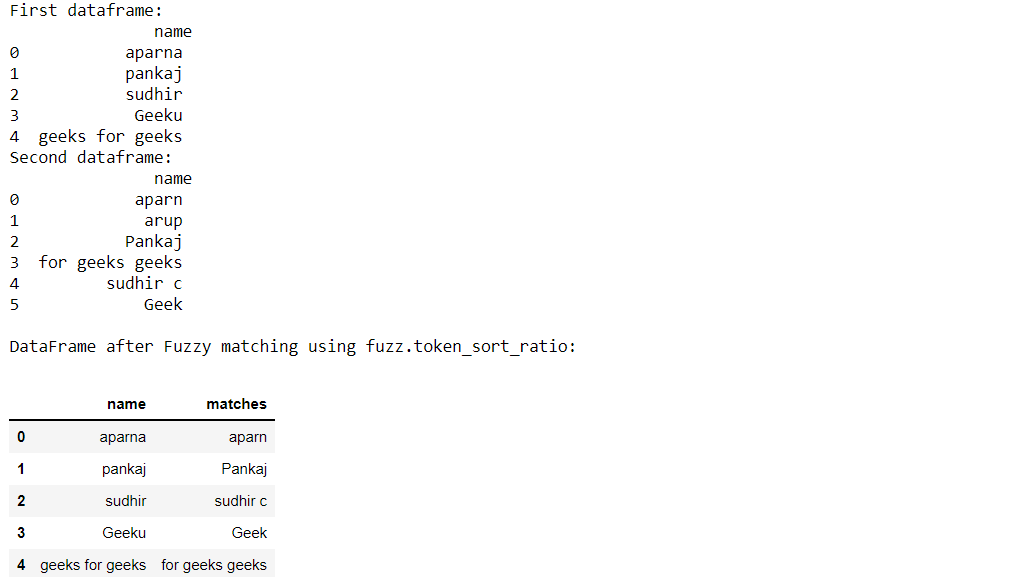Example 6: Using fuzz.token_set_ratio()

## Python3

 `import` `pandas as pd``from` `fuzzywuzzy ``import` `fuzz``from` `fuzzywuzzy ``import` `process`` ` `# creating the dictionaries``dict1 ``=` `{``'name'``: [``"aparna"``, ``"pankaj"``, ``"Geeku"``, ``                  ``"geeks for geeks"``]}`` ` `dict2 ``=` `{``'name'``: [``"aparn"``, ``"arup"``, ``"Pankaj"``,``                  ``"geeks for for geeks"``, ``                  ``"geeks for geeks"``, ``"Geek"``]}`` ` `# converting to pandas dataframes``dframe1 ``=` `pd.DataFrame(dict1)``dframe2 ``=` `pd.DataFrame(dict2)`` ` `# empty lists for storing the matches``# later``mat1 ``=` `[]``mat2 ``=` `[]``p ``=` `[]`` ` `# printing the pandas dataframes``print``(``"First dataframe:\n"``, dframe1,``      ``"\nSecond dataframe:\n"``, dframe2)`` ` `# converting dataframe column ``# to list of elements``# to do fuzzy matching``list1 ``=` `dframe1[``'name'``].tolist()``list2 ``=` `dframe2[``'name'``].tolist()`` ` `# taking the threshold as 80``threshold ``=` `80`` ` `# iterating through list1 to extract``# it's closest match from list2``for` `i ``in` `list1:``    ``mat1.append(process.extractOne(``      ``i, list2, scorer``=``fuzz.token_set_ratio))``dframe1[``'matches'``] ``=` `mat1`` ` `# iterating through the closest matches``# to filter out the maximum closest match``for` `j ``in` `dframe1[``'matches'``]:``    ``if` `j[``1``] >``=` `threshold:``        ``p.append(j[``0``])``    ``mat2.append(``","``.join(p))``    ``p ``=` `[]`` ` ` ` `# storing the resultant matches back ``# to dframe1``dframe1[``'matches'``] ``=` `mat2``print``(``"\nDataFrame after Fuzzy matching using token_set_ratio():"``)``dframe1`

Output: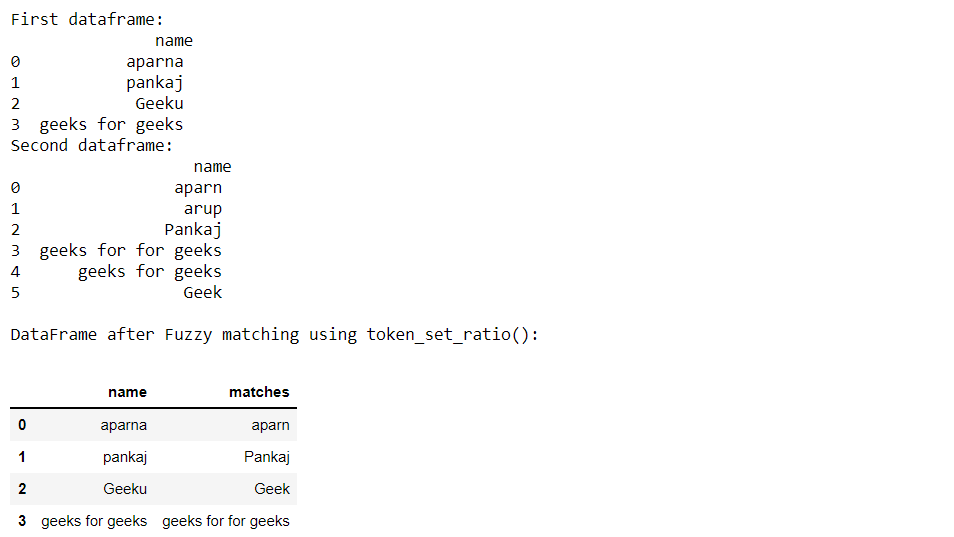My Personal Notes arrow_drop_up